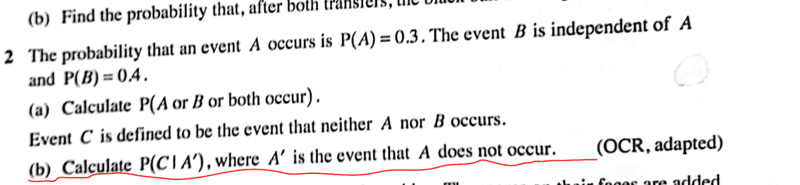# Calculate ##P(C|A')## in the given probability problem

Gold Member
Homework Statement:
See attached ( textbook question).
Relevant Equations:
Understanding of conditional probabilityMy interest is on part ##b## only. We know that ##A## and ##B## are independent and not mutually exclusive events therefore,

##P(C)=0.7×0.6=0.42##

##P(C|A')=\dfrac{P(C)-P(A∩C)}{P(A')}=\dfrac{0.42-(0.3×0.42)}{0.7}=\dfrac{0.294}{0.7}=0.42## which is wrong according to textbook solution.

Where is my mistake? cheers.

#### Attachments

Last edited by a moderator:

Homework Helper
Gold Member
Revisit what the value of Probability of "A intersect C" is.

Are A and C independent?

•chwala
Gold Member
@scottdave wawawawawawa this was a nice one man! Phew. Seen it...

#### Attachments

•scottdave